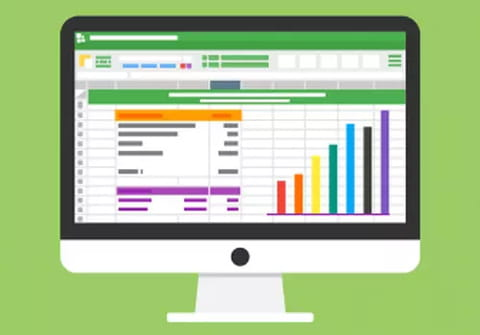# How to calculate VAT in Excel: formulaIn this article we will take a quick look at simple formulas in Excel, especially the calculation of VAT and inclusive of the sale price (including tax) for a purchase price without VAT (tax).

## Method

• Double click Excel icon on the desktop or the Start menu => All Programs.

• A new Excel document appears: we will make a summary invoice of purchase of the computer company Kioskea.

• The company bought: 4 PCs for 395.75€, 2 Phones for 869.50€, 6 USB for 22.50€, 4 flat screens for 315.00€ and 4 memory arrays for 58.00 €. We will put the result of Total Cost HT, for example, on cell D13.

• So, for this example we'll use the function: =SUM (B13 * C13)

• Explanation: = sum (unit price multiplied ht (*) amount), then click on keyboard input.

• Apply the formula: click on cell D13 (in our example)and then go to the bottom right of the cell till the black square, cursor will turn into a black cross. Hold the left mouse button and drag the handle until the cell D17 release, then we have the result of Totals HT.

• Calculate the VAT: we will put on cell E13, for example, the result of VAT. So, enter the formula =SUM (D13 * 19.6%), and then click on keyboard input and follow the same procedure as in the previous step.

• Calculation of VAT price for each item : We will put on cell F13 the result of price Taxes.

• 1st solution: enter the formula =SUM(D13: E13).
• 2nd solution: use the tool AutoSum and select the cell to add.
• Calculation of Total VAT invoice: we will put on cell F18 (in our example) the result of Total Taxes. Enter the formula =SUM(F13: F17).

any more excel questions? check out our forum!

Subject Replies